# ISEE Middle Level Quantitative : Probability

## Example Questions

← Previous 1 3 4

### Example Question #1 : Data Analysis And Probability

Two fair six-sided dice are thrown. What is the probability that the product is greater than or equal to 20?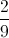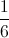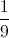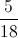Explanation:

The rolls that yield a product greater than or equal to 20 are: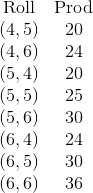These are 8 out of 36 rolls, so the probability of getting one of them is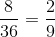### Example Question #1 : How To Find The Probability Of An Outcome

Using the information given in each question, compare the quantity in Column A to the quantity in Column B.

A pair of regular dice (with sides numbered from 1-6) is thrown.

Column A                       Column B

the odds of                     the odds of

rolling a total of 8          rolling a total of 7

The quantity in Column A is greater.

The relationship cannot be determined from the information given.

The quantity in Column B is greater.

The two quantities are equal.

The quantity in Column B is greater.

Explanation:

Consider the different ways in which you could roll an 8 or a 7.

You could roll 8 by the following combinations:

2-6, 3-5, 4-4, 5-3, 6-3

so the odds of rolling 8 are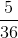.

You could roll 7 by the following combinations:

1-6, 2-5, 3-4, 4-3, 5-2, 6-1

so the odds of rolling 7 are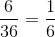.

The answer, therefore, is that Column B is greater.

### Example Question #3 : Data Analysis And Probability

A standard deck of fifty-two cards is altered by removing the tens and replacing them with the queens from another deck. A card is drawn at random from the altered deck.

Which is the greater quantity?

(a) The probability that the card is a face card

(b)Note: a face card is a jack, a queen, or a king.

(a) is greater

(b) is greater

(a) and (b) are equal

It is impossible to tell from the information given

(b) is greater

Explanation:

With the replacement of the tens with the queens, there are still 52 cards in the deck, but now, there are four jacks, eight queens, and four kings - 16 face cards. The probability that a random card is a face card is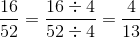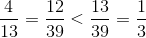### Example Question #4 : Data Analysis And Probability

A standard deck of fifty-two cards is altered by removing the black aces. A card is drawn at random from the altered deck.

Which is the greater quantity?

(a) The probability that a face card will be drawn

(b)Note: a face card is a jack, a queen, or a king.

It is impossible to tell from the information given

(b) is greater

(a) is greater

(a) and (b) are equal

(b) is greater

Explanation:

The removal of the two black aces leaves a deck of 50 cards, with all 12 face cards remaining. The probability that a randomly drawn card is a face card is therefore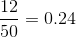Since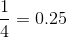, the probability is less than.

### Example Question #5 : Data Analysis And Probability

Sandy has two red dice; Tommy has one blue die. All three dice are fair.

Sandy rolls her red dice once and notes her sum. Tommy rolls his blue die twice and notes his sum.

Which is the greater quantity?

(a) The probability that Sandy will roll a seven or higher

(b) The probability that Tommy will roll a seven or higher

(a) and (b) are equal

It is impossible to tell from the information given

(a) is greater

(b) is greater

(a) and (b) are equal

Explanation:

Since each die is fair, the roll of each die is an independent event; also, the second roll of the blue die is independent of the first roll. Therefore, the probabilities of each outcome are the same for Sandy rolling two dice simultaneously as for Tommy rolling one twice. (a) and (b) are equal.

### Example Question #6 : Data Analysis And Probability

A standard deck of fifty-two cards is altered by removing the jacks and replacing them with the queens from another deck. A card is drawn at random from the altered deck.

Which is the greater quantity?

(a) The probability that a black card is drawn

(b) The probability that a red card is drawn

(a) is greater

It is impossible to tell from the information given

(b) is greater

(a) and (b) are equal

(a) and (b) are equal

Explanation:

The removal of the jacks removes two black cards and two red cards from the deck; replacement with the four queens from another deck adds two black cards and two red cards. The number of black cards and the number of red cards remain the same, so the probabilities remain equal.

### Example Question #7 : Data Analysis And Probability

Two fair dice are thrown. Which is the greater quantity?

(a) The probability that the sum wiil be 5 or less

(b)(b) is greater

(a) and (b) are equal

It is impossible to tell from the information given

(a) is greater

(a) is greater

Explanation:

For the sum of the dice to be 5 or less, one of the following rolls must be thrown:

(1,1). (1,2), (2,1), (1,3), (2,2), (3,1), (1,4), (2,3), (3,2), (4,1)

This makes 10 out of 36 rolls. Since one-fourth of 36 is 9, the probability of throwing a 5 or less is greater than.

### Example Question #8 : Data Analysis And Probability

A card is drawn at random from a deck of 53 cards - the standard deck including the joker. Which is the greater quantity?

(a) The probability of drawing a black card

(b)(b) is greater

(a) and (b) are equal

It is impossible to tell from the information given

(a) is greater

(b) is greater

Explanation:

26 of the 53 cards are black (the joker counts as neither).

Half of 53 is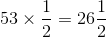26 is less than this, so black cards comprise less than half the deck, and the probability of drawing a black card is less than.

### Example Question #9 : Data Analysis And Probability

There is a bag with 10 yellow markers, 3 red markers, 2 blue markers, and 5 green markers. What is the probability of picking a marker that is not red?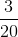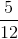Explanation:

Probability involves part over whole. Therefore, you must find the total number of markers, which is 20. Then, combine all of the markers that are not red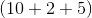, which gives you 17. Put 17 over 20 to get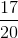### Example Question #10 : Data Analysis And Probability

Two standard decks of fifty-two cards, one with a green backing and one with a blue backing, are presented to you. The green deck has all of its hearts removed; the blue deck is left complete. Which is the greater quantity?

(A) The probability that a card randomly drawn from the green deck is a two

(B) The probability that a card randomly drawn from the blue deck is a two

(B) is greater

(A) and (B) are equal

It is impossible to determine which is greater from the information given

(A) is greater

The green deck, having had all of its hearts removed, has thirty-nine cards; one of the hearts removed is a two, so there are three of the four twos left. The probability of a card drawn from the green deck being a two is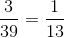.
The blue deck, being complete, has fifty-two cards, including all four of its twos. The probability of a card drawn from the blue deck being a two is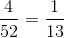.